# Constructions

## Perpendicular bisectors of a line

There are several constructions that you will need to be able to perform. Full explanations about how to perform them are on the videos here.

The perpendicular bisector of a line is a line that is at an exact right angle to another line.

Step 1: Set your compass to slightly more than half way over the line

Step 2: Place your compass on the edge of the line

Step 3: Draw arcs above and below the line

Step 4: Move the compass to the other end of the line and draw arcs again

Step 5: With a pencil and a ruler join the points where the arcs cross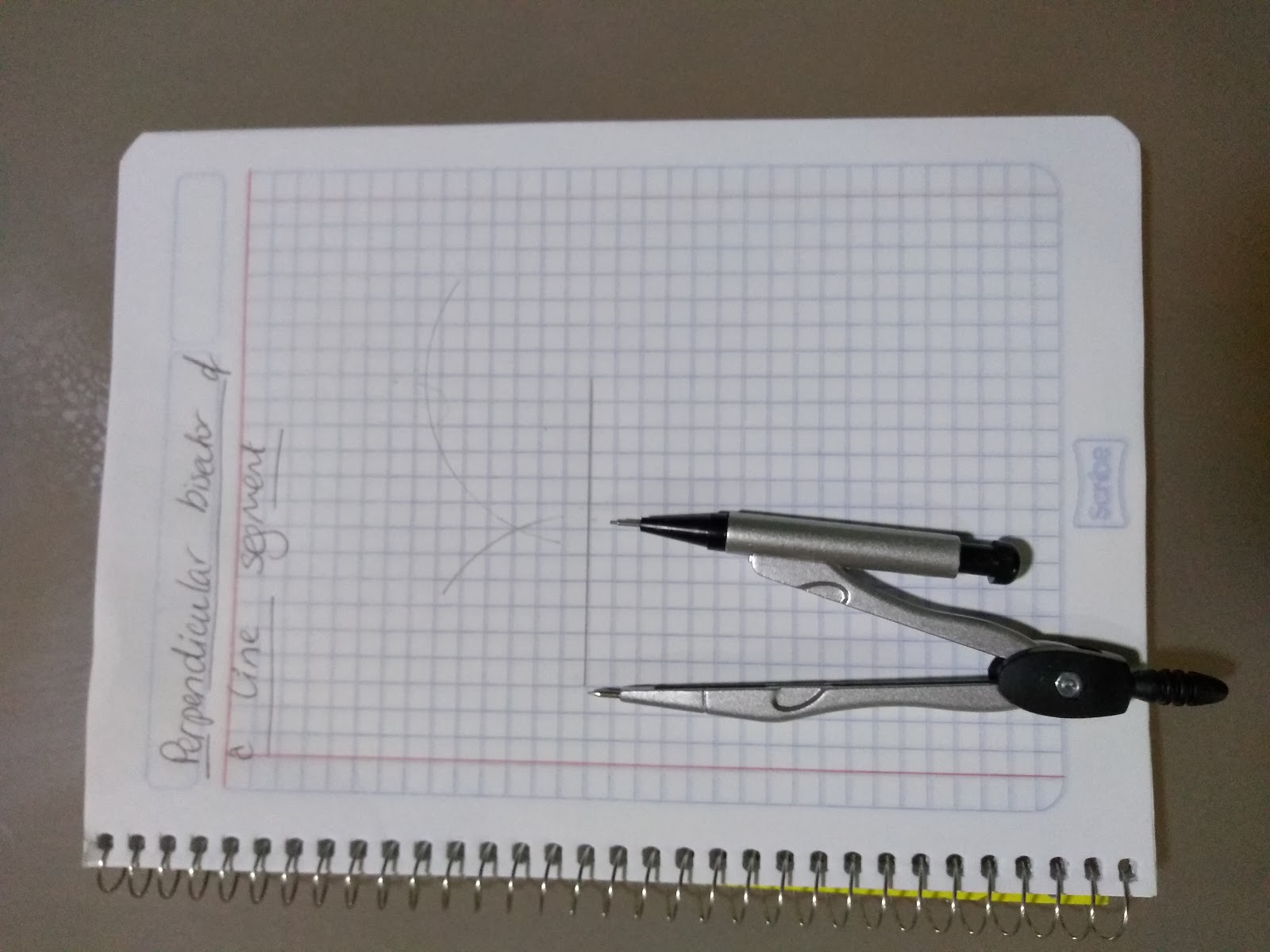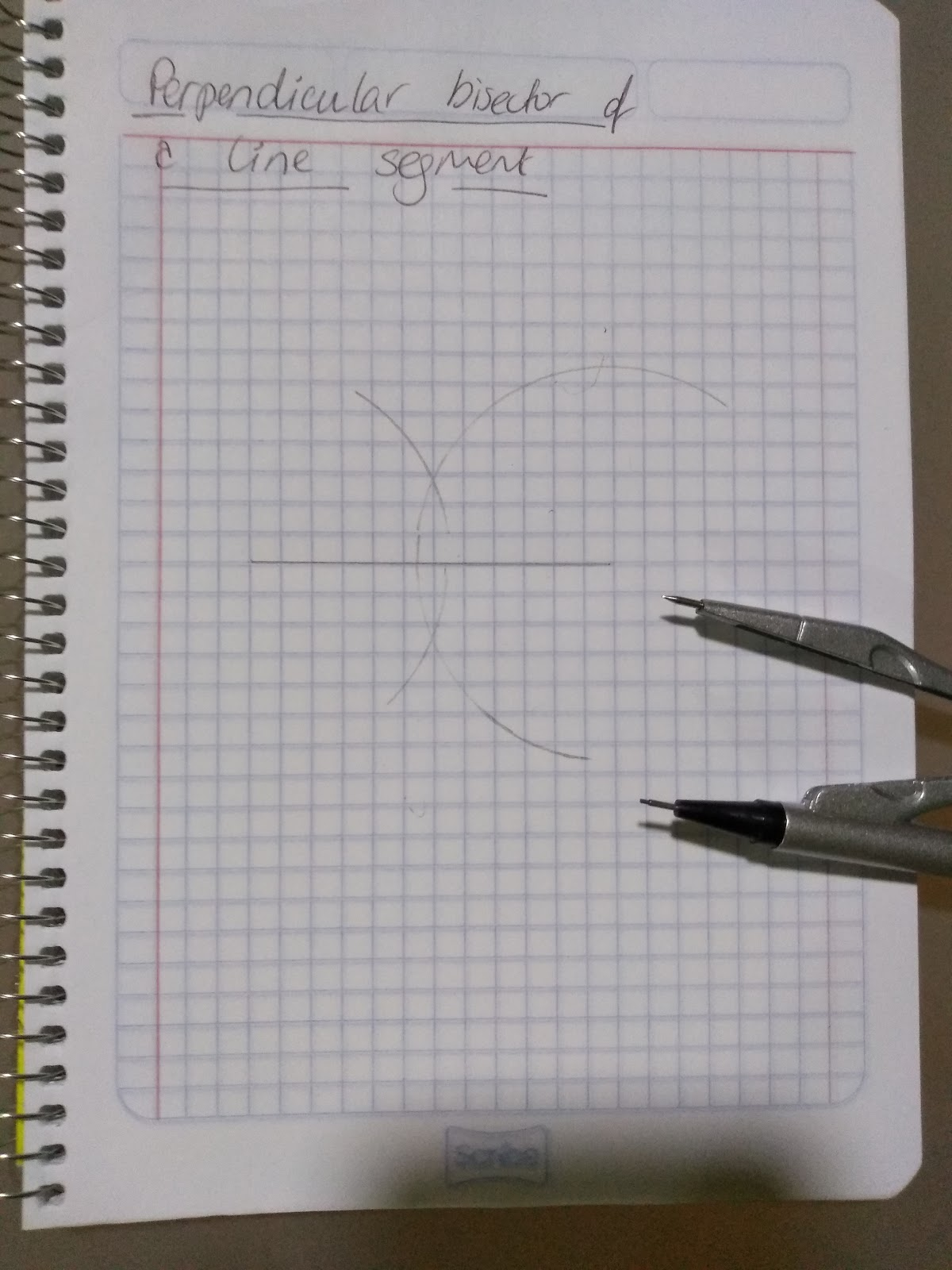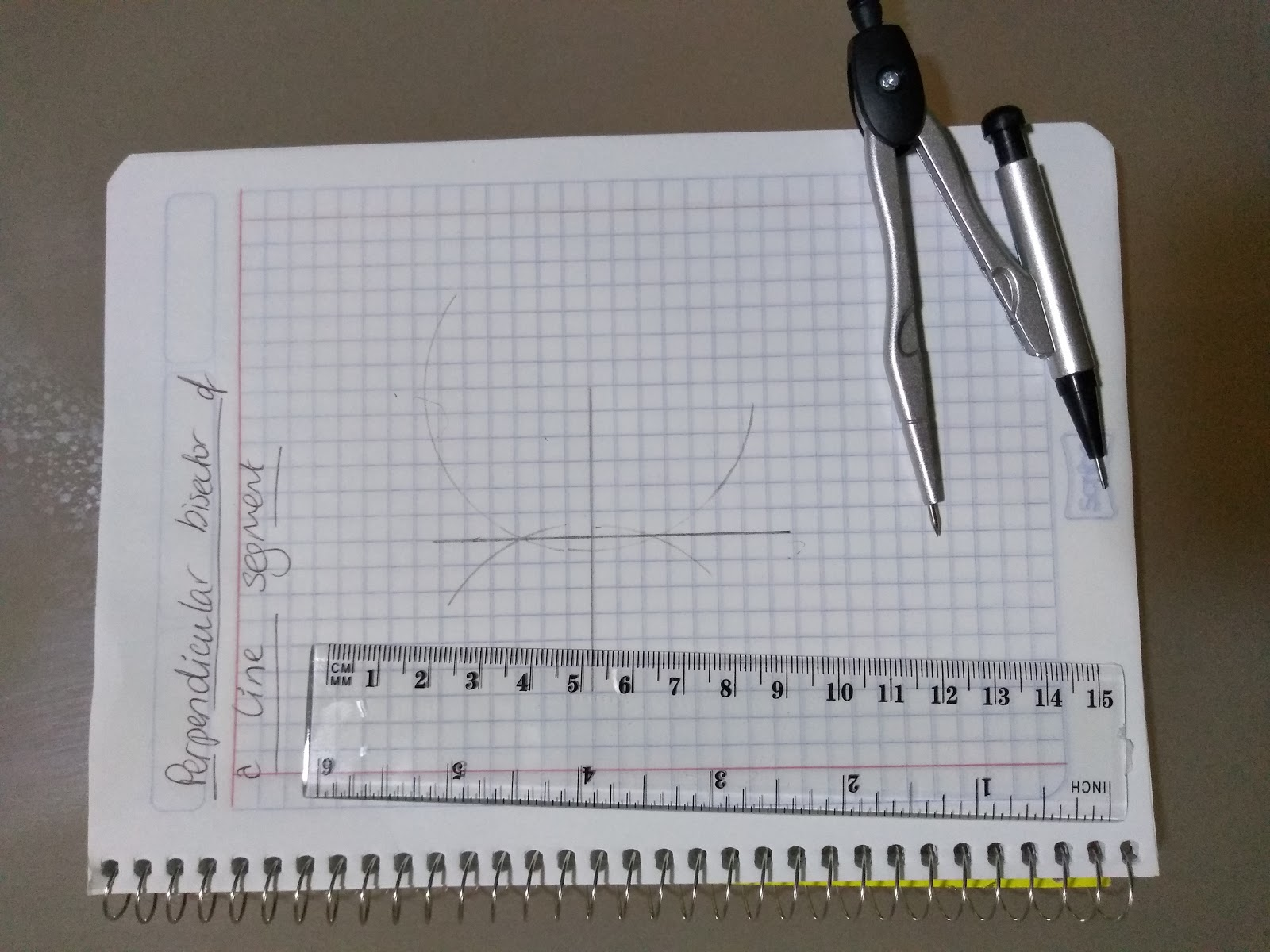## Constructing a perpendicular bisector

Step 1: Put your compass on the point ( P below) and create an arc that crosses the line.

Step 2: Move your compass onto the point where it has crossed the line then draw an arc

Step 3: Move your compass onto the point where the arc in Step 2 crosses the line and draw another arc

Step 4: Draw a line from the point P to the point where the arcs cross.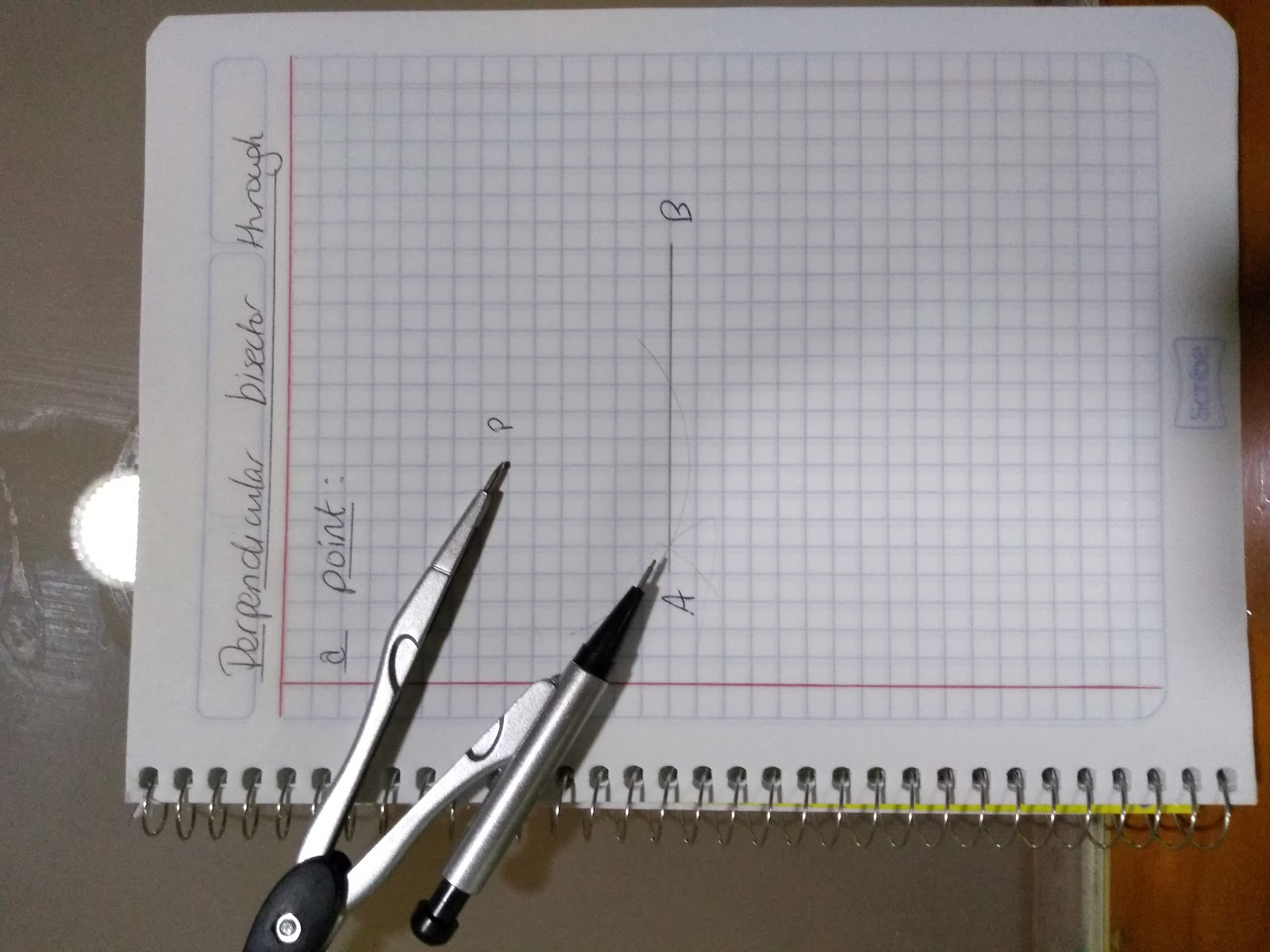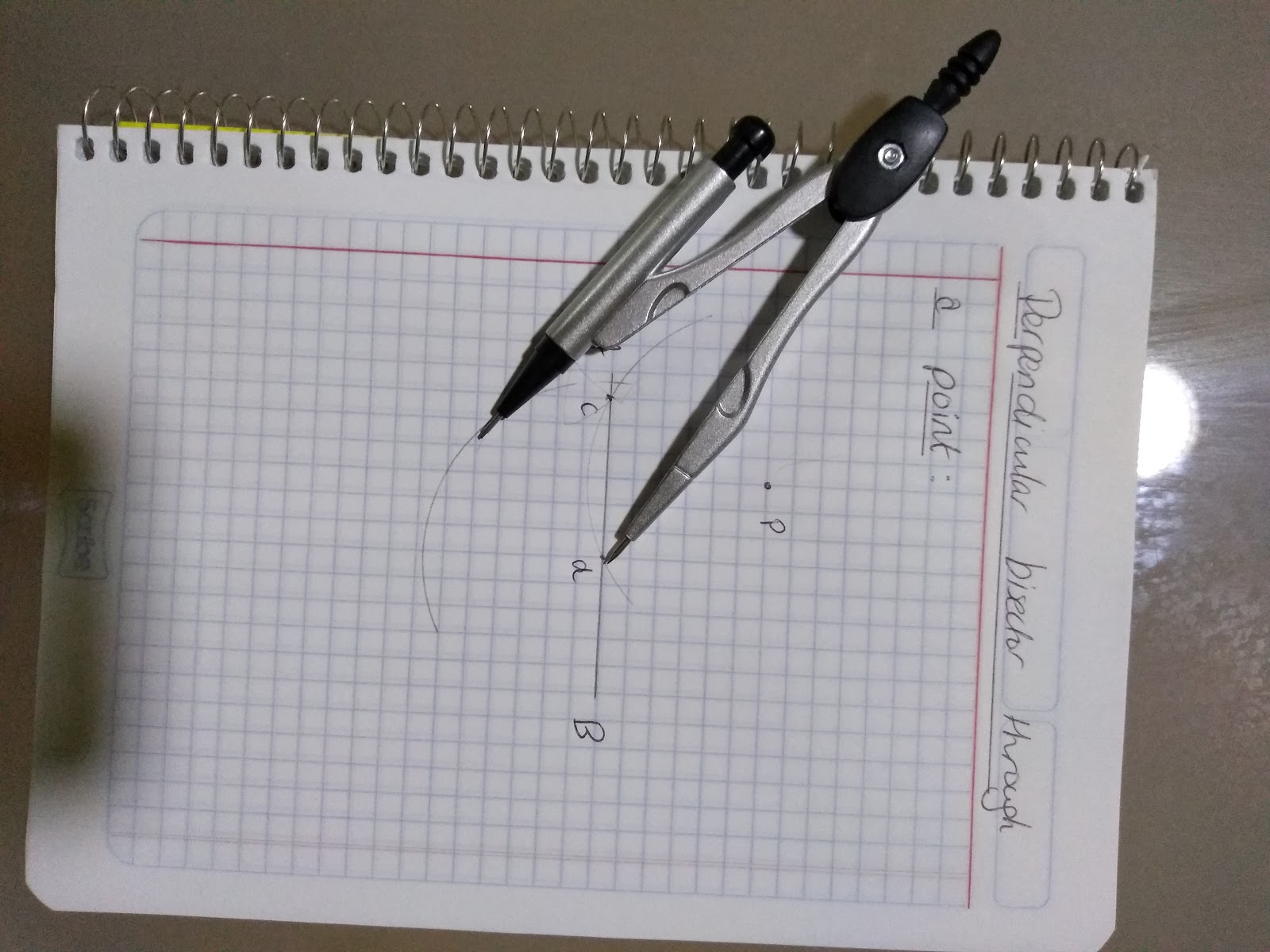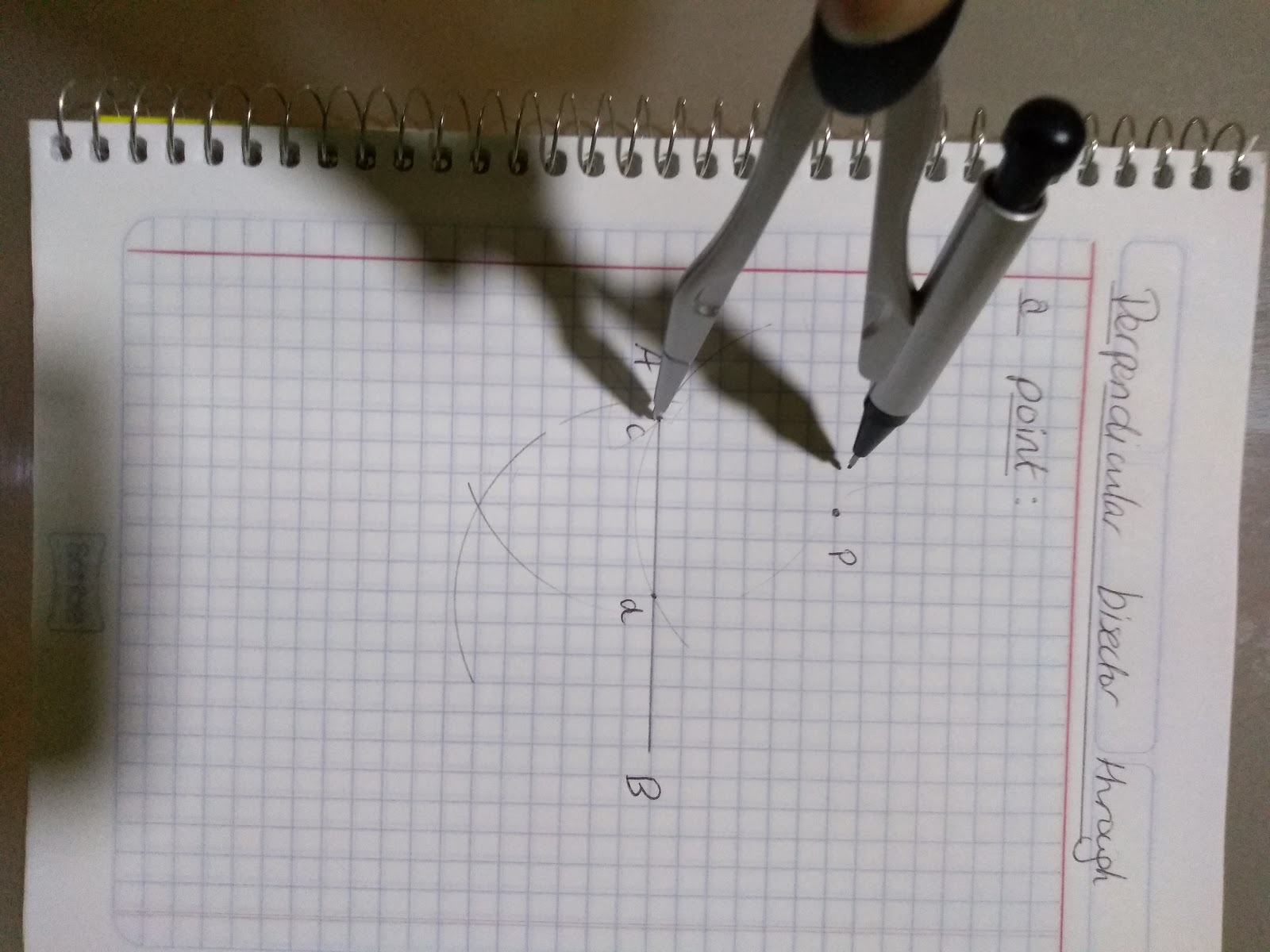## Loci Problems

You can use these constructions to help you solve loci problems!

A common problem is to construct a loco around a line to do this you need to use a compass and a ruler.

Firstly, draw two secs around the ends of the line:In this case we want a loci of 2 squares, so we make sure the compass is set to that amount.

We then complete the loci by drawing two straight lines, two squares away from the original line.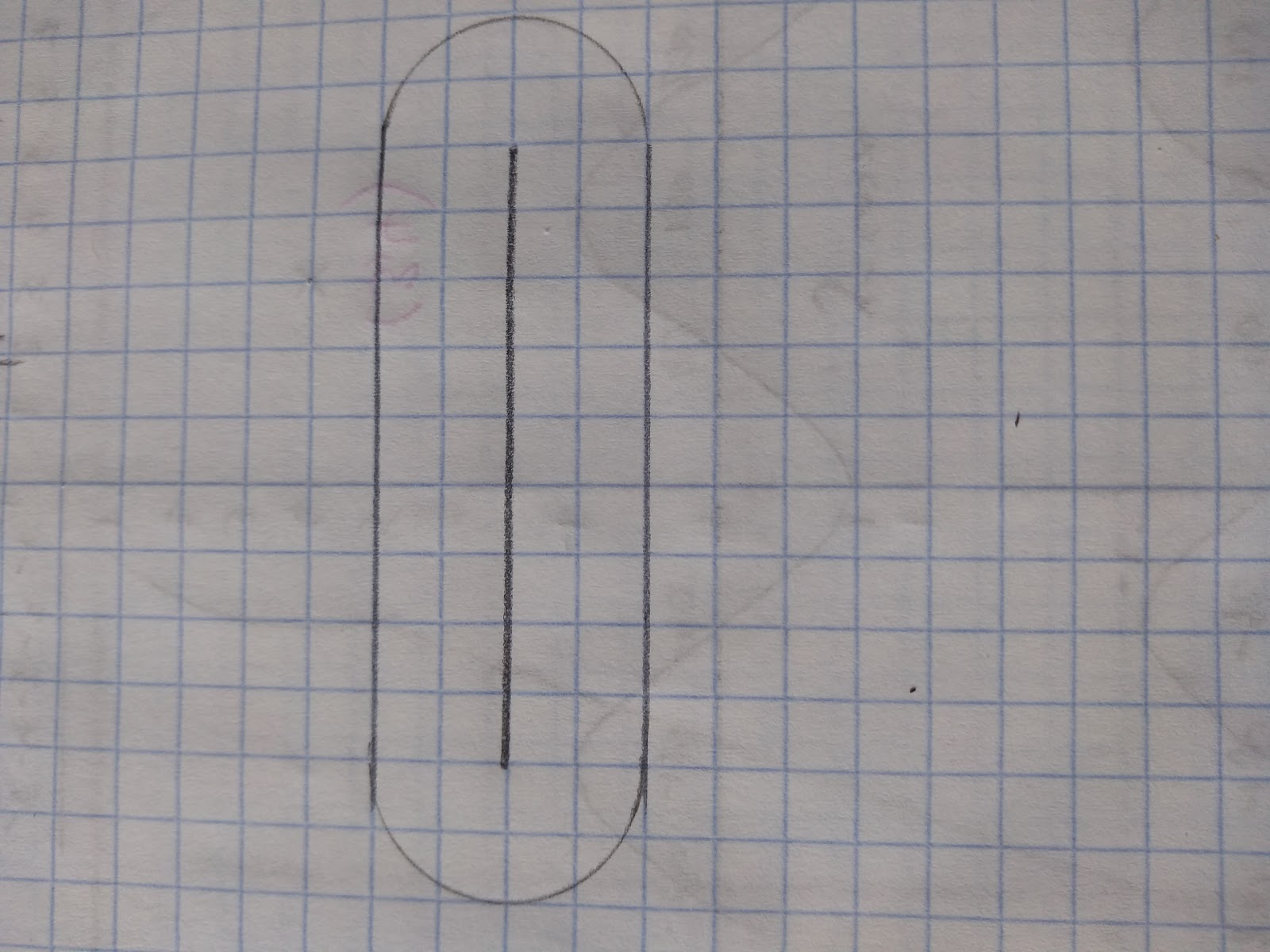Now we have our loci!

## Perpendicular Distances

The perpendicular distance from a point to a line is the shortest distance to the line.

What shape can you draw exactly to find angles of 60 degrees?
Triangle